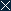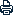Home | Sitemap | Contact | Chinese | CAS
 Search:
 About AMSS Research People International Cooperation News Societies & Journals Papers Resources Education & Training Join Us LinksLocation: Home >  Research >  Research Progress
 $L^2$ Decay of Solutions to a Micro-Macro Model for Polymeric Fluids Near Equilibrium
 Author：
 ArticleSource：
 Update time： 2009-07-22CloseA A APrint

# $L^2$ Decay of Solutions to a Micro-Macro Model for Polymeric Fluids Near Equilibrium

### SIAM J. Math. Anal. Volume 40, Issue 5, pp. 1905-1922 (2009)

Published January 14, 2009

In this paper, we consider the long time decay of the $L^2$ norm to the global solutions $(u,\psi)$ constructed in [F.-H. Lin, C. Liu, and P. Zhang, Comm. Pure Appl. Math., 60 (2007), pp. 838–866] for a micro-macro model of polymeric fluids near equilibrium $(0,M)$. Under the additional assumption that $u_0\in\dot{H}^{-\kappa}(\mathbf{R}^3)$, $(\psi_0-M)/\sqrt{M}\in L^{2} (\mathbf{R}^3_q;\dot{H}^{-\kappa}(\mathbf{R}^3_x))$, we prove that $(u(t),\psi(t))$ tends to $(0,M)$ as $t$ goes to infinity with decaying rate $\|u(t)\|_{L^2}\leq C(1+t)^{-\frac{b}{2}}$ and $\|\frac{\psi(t)-M}{\sqrt{M}}\|_{L^2}\leq C(1+t)^{-\frac{b+1}{2}}$ for $b=\min(\kappa,\frac{3}{2})$. In general, without this additional assumption, we shall present an explicit long time decaying formula for $\|u(t)\|_{L^2}$ and $\|\frac{\psi(t)-M}{\sqrt{M}}\|_{L^2}$.

©2009 Society for Industrial and Applied Mathematics
 Copyright@2008, All Rights Reserved, Academy of Mathematics and Systems Science, CAS Tel: 86-10-82541777 Fax: 86-10-82541972 E-mail: contact@amss.ac.cn